### Autocorrelation Weights

In general, the choice of a weighting scheme is subjective. You can obtain different results by using different schemes, options, and parameters. PROC VARIOGRAM offers you considerable flexibility in choosing weights that are appropriate for prior considerations such as different hypotheses about neighboring areas, definition of the neighborhood structure, and accounting for natural barriers or other spatial characteristics; see the discussion in Cliff and Ord (1981, p. 17). As stressed for all types of spatial analysis, it is important to have good knowledge of your data. In the autocorrelation statistics, this knowledge can help you avoid spurious correlations when you choose the weights.

The starting point is to assign individual weights to each one of thedata values,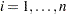, with respect to the rest. An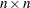matrix of weights is thus defined, such that for any two locations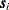and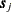, the weight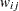denotes the effect of the valueat locationon the valueat location. Depending on the nature of your study, the weightsneed not be symmetric; that is, it can be true that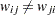.

#### Binary and Nonbinary Weights

The weightscan be either binary or nonbinary values. Binary values of 1 or 0 are assigned if the SRF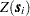at one locationis deemed to be connected or not, respectively, to its value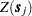at another location. Nonbinary values can be used in the presence of more refined measures of connectivity between any two data points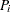and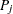. PROC VARIOGRAM offers a choice between a binary and a distance-based nonbinary weighting scheme.

In the binary weighting scheme the weight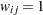if the data pair atandis closer than the user-defined distance that is defined by the LAGDISTANCE= option, and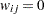if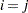or in any other case. For that reason, in the COMPUTE statement, if you specify the WEIGHTS=BINARY suboption of the AUTOCORRELATION option when the NOVARIOGRAM option is also specified, then you must also specify the LAGDISTANCE= option.

The nonbinary weighting scheme is based on the pair distances and is invoked with the WEIGHTS=DISTANCE suboption of the AUTOCORRELATION option. PROC VARIOGRAM uses a variation of the Pareto form functional to set the weights. Namely, the autocorrelation weight for every point pairandlocated atand, respectively, is defined as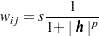where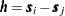and
and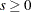are user-defined parameters for the adjustment of the weights.

In particular, the power parameteris specified in the POWER= option of the DISTANCE suboption within the AUTOCORRELATION option. The default value for this parameter is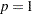. Also, the scaling parameteris specified by the SCALE= option in the DISTANCE suboption of the AUTOCORRELATION option. The default value for the scaling parameter is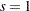. You can use theandparameters to adjust the actual values of the weights according to your needs. Variations in the scaling parameterdo not affect the computed values of the Moran’sand Geary’sautocorrelation coefficients that are introduced in the section Autocorrelation Statistics Types.

#### Nonbinary Weights with Normalized Distances

PROC VARIOGRAM offers additional flexibility in the DISTANCE weighting scheme through an option to use normalized pair distances. You can invoke this feature by specifying the NORMALIZE option in the DISTANCE suboption of the AUTOCORRELATION option. In this case, the distances used in the definition of the weights are normalized by the maximum pairwise distance(see the section Computation of the Distribution Distance Classes and Figure 98.24); the weights are then defined as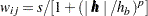.

Most likely,has a different value for different data sets. Hence, it is suggested that you avoid using the weights you obtain from the preceding equation and one data set for comparisons with the weights you derive from different data sets.

#### Symmetric and Asymmetric Weights

The weighting schemes presented in the preceding paragraphs are symmetric; that is,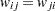for every data pair at locationsand. However, you can also define asymmetric weights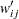such that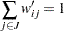for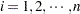, where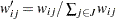,. In the distance-based scheme,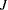is the set of all locations that form point pairs with the point at. In the binary scheme,is the set of the locations that are connected tobased on your selection of the LAGDISTANCE= option; see Cliff and Ord (1981, p. 18). The weightsare row-averaged (or standardized by the count of their connected neighbors). You can apply row averaging in weights when you specify the ROWAVG option within either the BINARY or DISTANCE suboptions in the AUTOCORRELATION option.